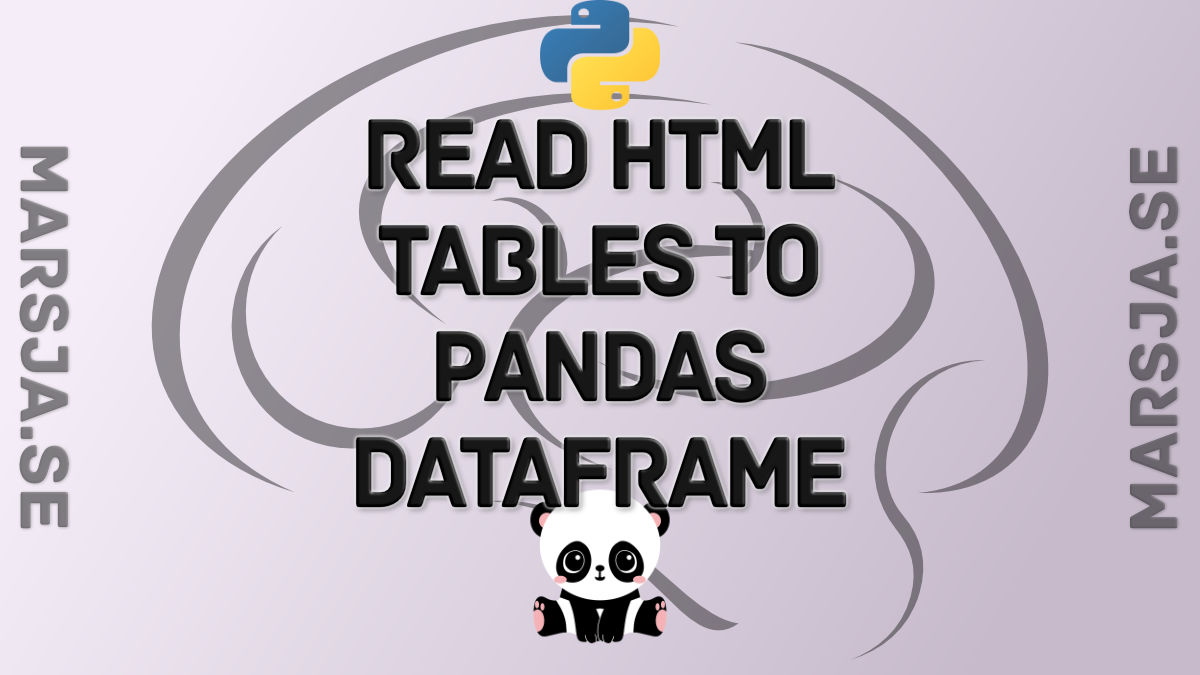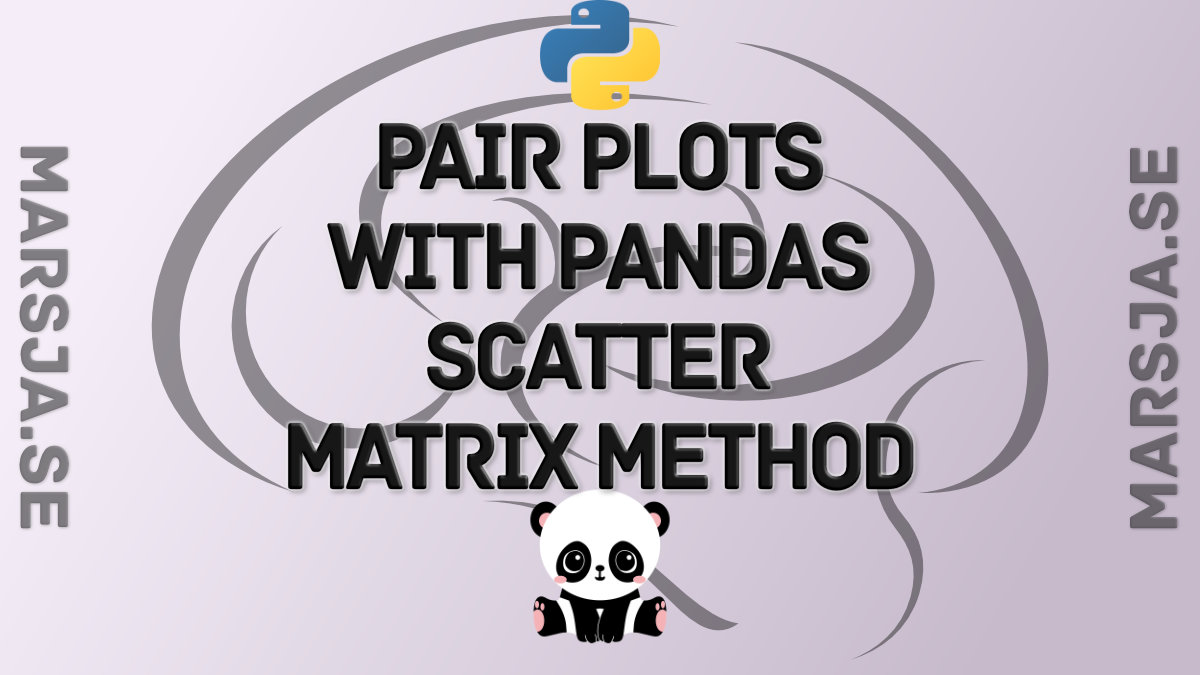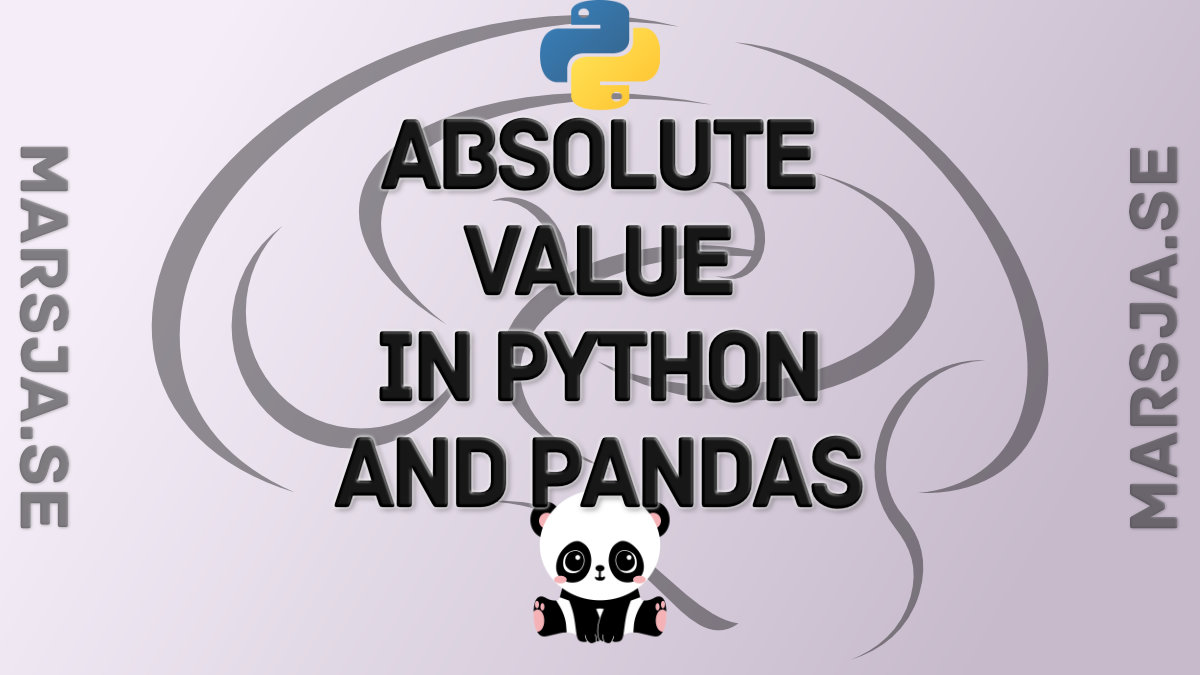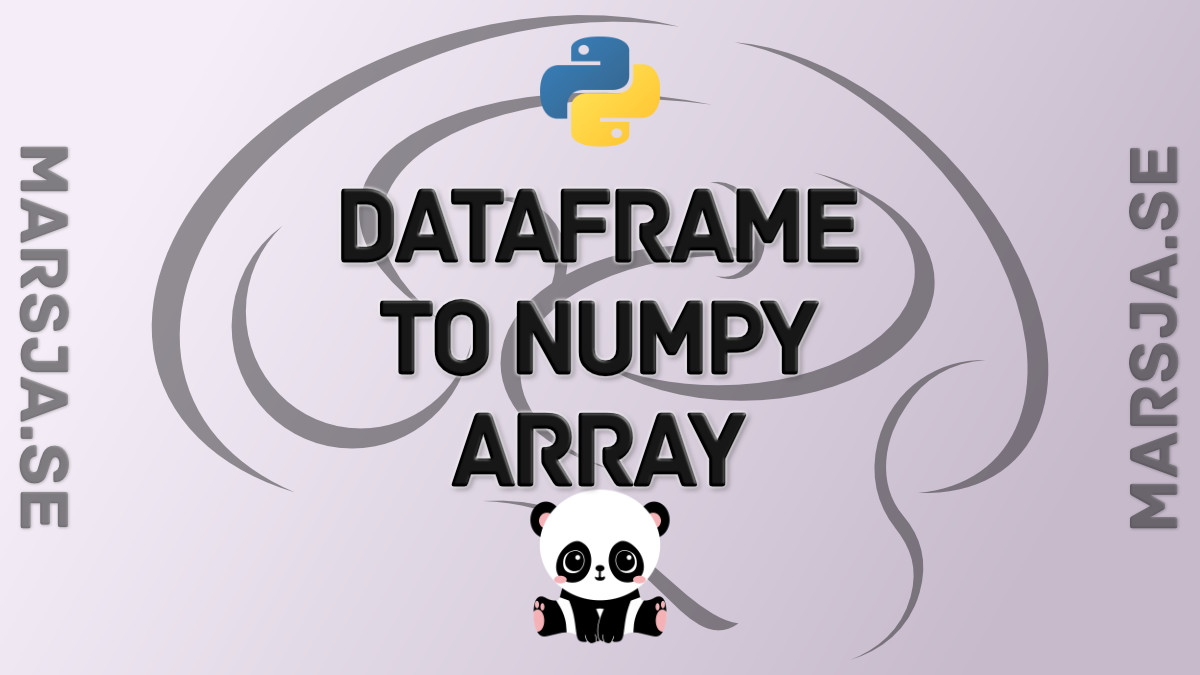## How to use Pandas read_html to Scrape Data from HTML Tables

In this Pandas tutorial, we will go through the steps on how to use Pandas read_html method for scraping data from HTML. First, in the simplest example, we are going to use Pandas to read HTML from a string. Second, we are going to go through a couple of examples in...## How to use Pandas Scatter Matrix (Pair Plot) to Visualize Trends in Data

In this Python data visualization tutorial, we will work with Pandas scatter_matrix method to explore trends in data. Previously, we have learned how to create scatter plots with Seaborn and histograms with Pandas, for instance. In this post, we’ll focus on scatter...## How to get Absolute Value in Python with abs() and Pandas

In this Python tutorial, we will learn how to get the absolute value in Python. First, we will use one of Pythons built-in functions abs() to do this. In this section, we will go through a couple of examples of how to get the absolute value. Second, we will import...## How to Convert a Pandas DataFrame to a NumPy Array

In this short Python Pandas tutorial, we will learn how to convert a Pandas dataframe to a NumPy array.  Specifically, we will learn how easy it is to transform a dataframe to an array using the two methods values and to_numpy, respectively. Furthermore, we will...